Geometry Fundamentals

Seven $1 \times 1 \times 1$ unit cubes are glued together to create the figure shown, including one cube in the middle of the figure that you can't see.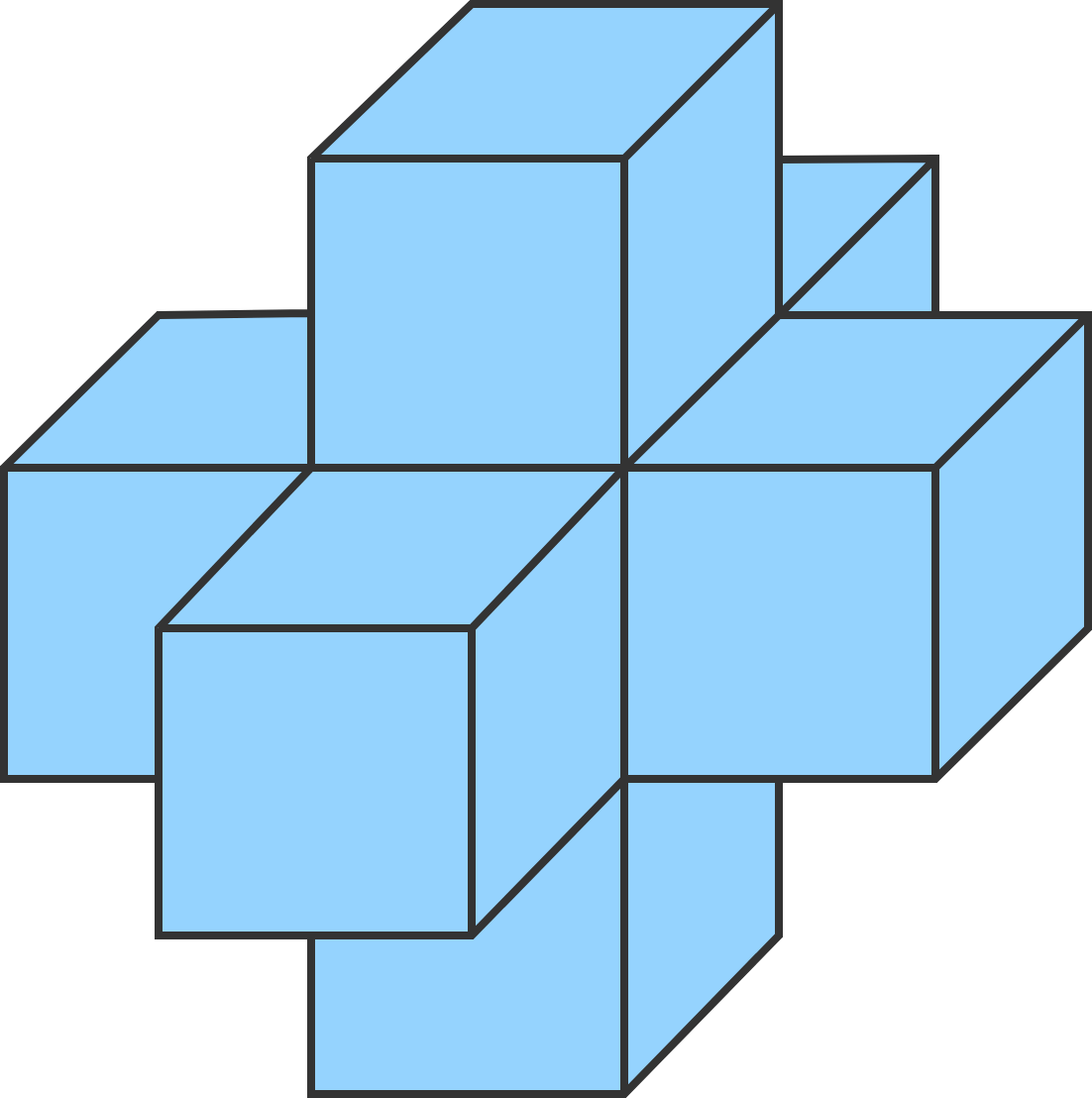What is the surface area of the figure?

Going 3D

Three sets of eight identical cubes are placed into the three different figures shown. Which figure has the greatest surface area?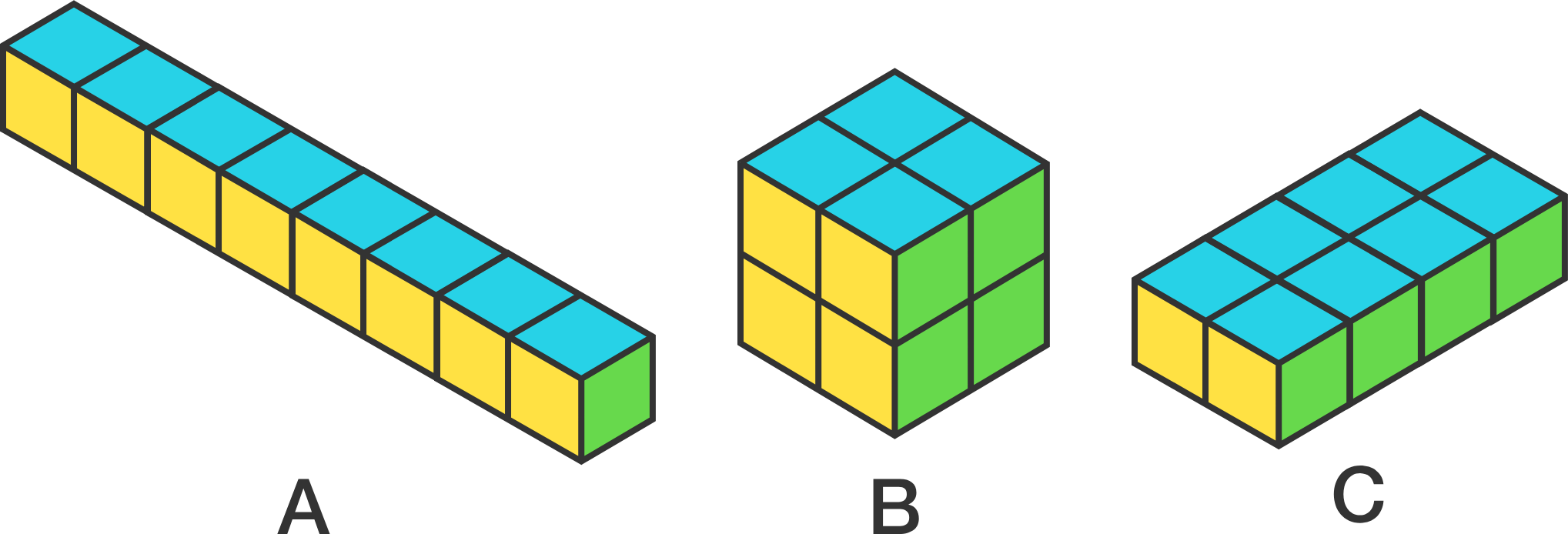Surface area is the total area of all of a 3D figure's surfaces.

Going 3D

27 unit cubes are packed together to create this $3\times 3\times 3$ cube. Each face of a unit cube has an area of 1.

If all 8 of the corner cubes are removed, by how much will the surface area of the figure increase in the structure that remains?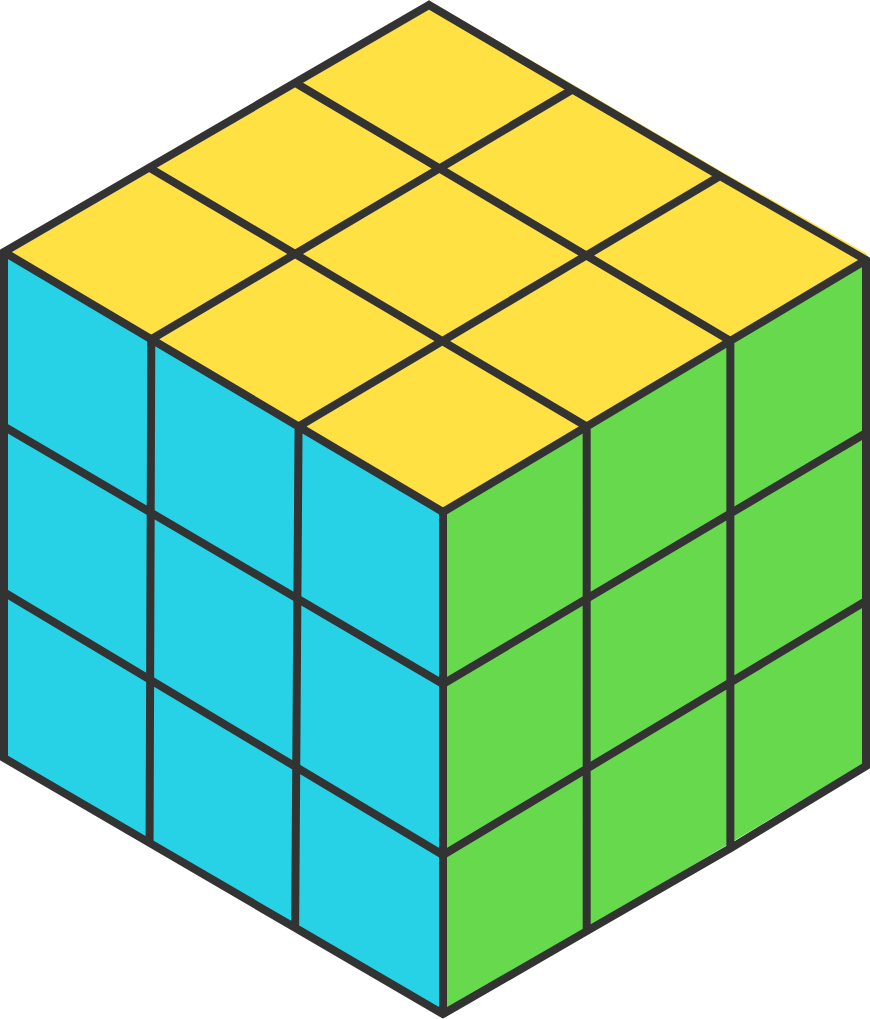Going 3D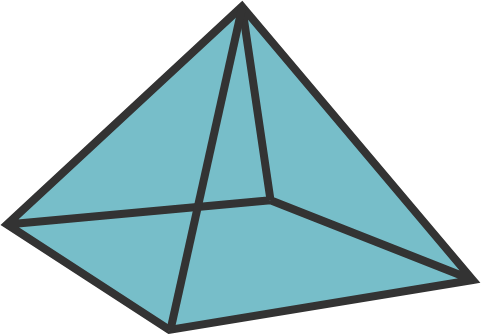How many of the following nets can fold up into the square pyramid shown above?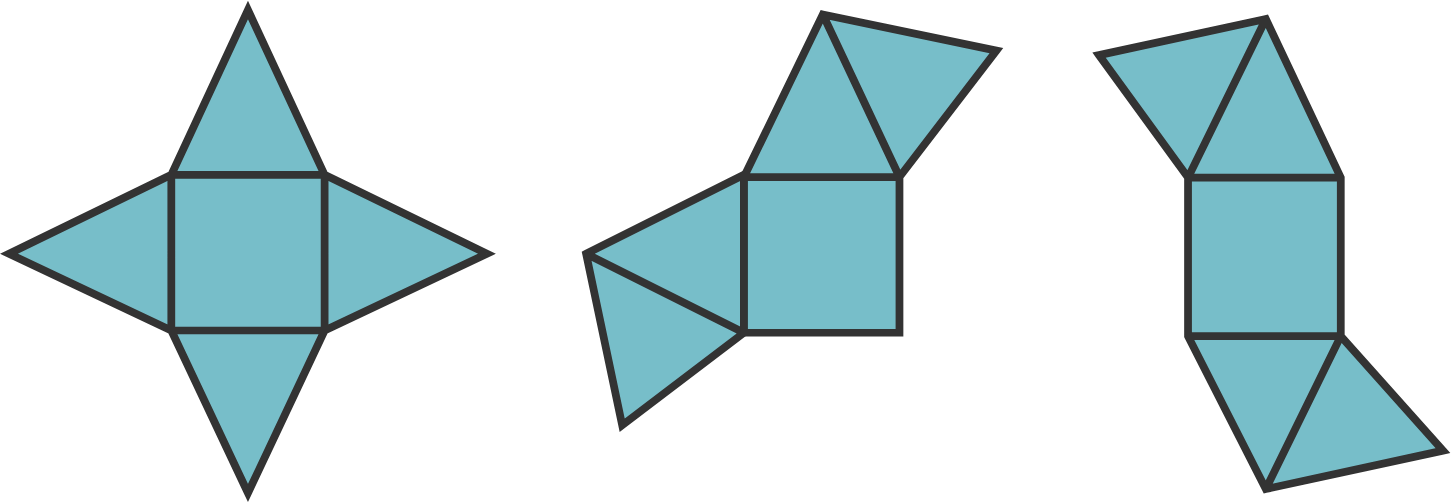Going 3D

An ant sits in the middle of a face of a solid cube that has side lengths of $3 \text{ cm}$. She must get to the exact same spot on the opposite face only by walking on the surface. Can she walk less than $6 \text{ cm}$?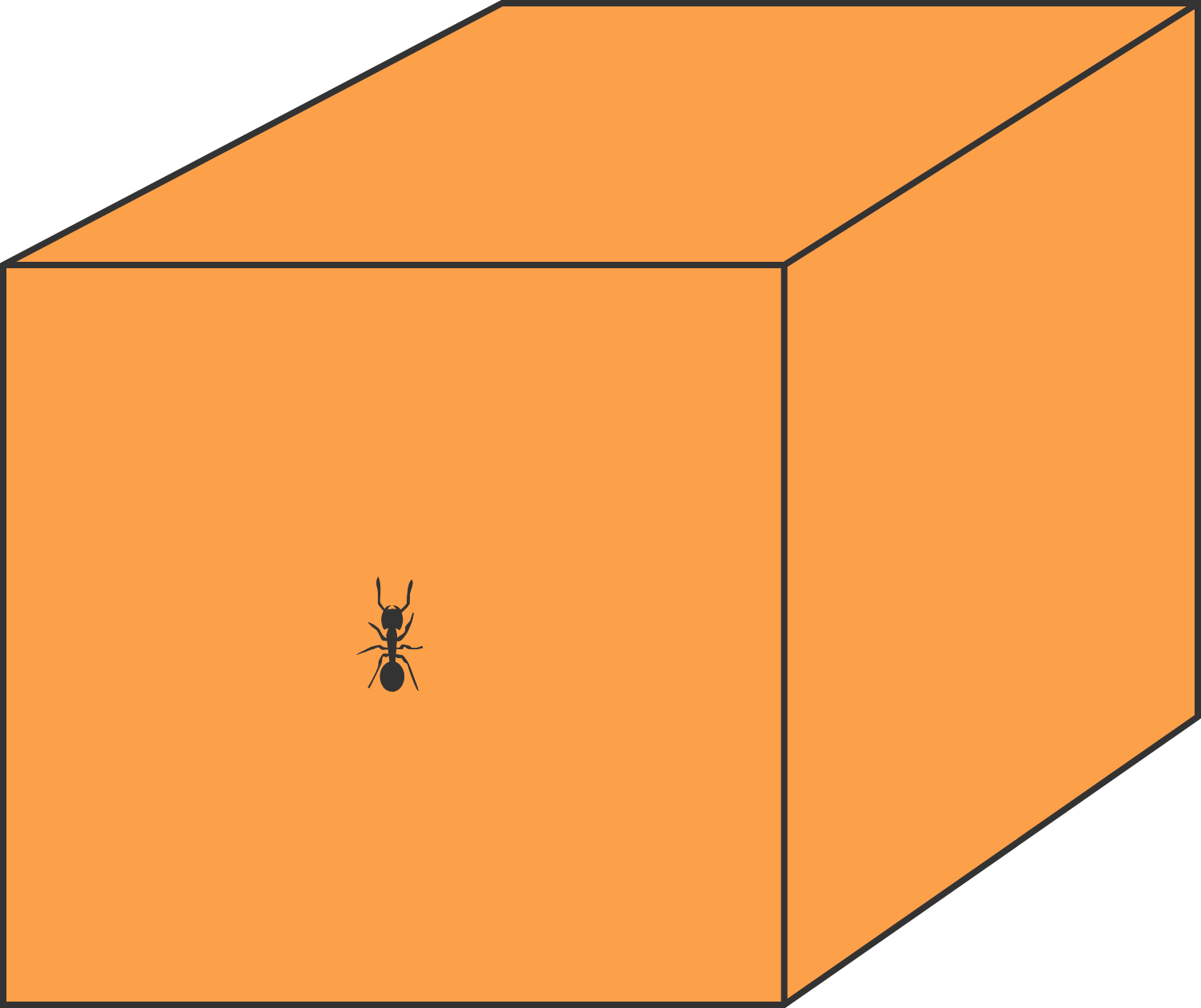×